top of page
Search

Updated: Apr 7, 2022

In my role as Tableau Doctor at a client, I was tasked with assisting a user in creating a ‘spider’ chart (otherwise known as a radar chart). The user had 20+ static dimension values and had been creating the chart in Excel previously.

I thought of a similar viz that a Cleartelligence colleague had created. In that case, points were plotted along the outer edges of a circle to represent the 12 months in a year. For the radar chart to work, though, I would have to come up with a way to plot the points on an axis from the center of the spider chart to the outer edge. When points are plotted at the outer edge of a circle the radius is 1 for all points (this radius can be changed if desired). I needed a custom radius that would plot the point relative to the min/max values of the relevant data. To do this, I used this formula: (Value-Minimum)/(Maximum-Minimum). For simplicity’s sake, let’s say the values in our dataset range from 0-100. 0 is our Min and 100 is our Max. To plot a point with a value of 80 the formula would be (80-0)/(100-0). The result is .80, and that is the radius for that point. Given that, the point will fall 4/5 of the way out from the center to the edge.

Before we move further along, here are a couple examples of what the end-result may look like. These can come in all shapes and sizes, so you can get creative!## Let’s go over the process for building these charts from start to finish.

In the spirit of Tom Brady winning his sixth Super Bowl championship, I had the idea of comparing him to current and future Pro Football Hall of Fame quarterbacks. I chose five total players, so theshape will end up being a pentagon. In some cases, you will have to specifically plan where to plot points. The idea is to split up the available 360 degrees in a circle. For the triangle, I used 0, 120, and 240 degrees. In that chart the x and y axis had to be flipped in Tableau because in the default orientation 0/360 sit at 3:00 (see image). In most cases, you can get away with simply dividing 360/# of dimensional values. Then for subsequent rows, do previous row + (360/# of dimensional values). The reason I did these angle calcs in the data prep stage here is because we need an extra row with the same data as the first row in order to connect the line in Tableau. If the extra row is not there, there will be a gap in the line. In a very small data set you can add this row in manually, but in a large dataset you would need to generate rows using Alteryx or custom SQL.

This is a sample of the Quarterbacks dataset I used showing two metrics. The degrees are split evenly five ways from a pie of 360°. This dashboard will have multiple statisticalcategories, so there has to be an extra row for each category. I leave the main dimension column (Player) blank so that when labeling there won’t be a duplicate that has to be deleted. The data is now ready for Tableau.

The first step in Tableau is to create the radius calculation which I wrote about in the first paragraph. We need a min and max value. Since there are multiple categories here, a Fixed LOD calc is necessary for both min and max. Radius could be done in one calculated field, but I will break out the min and max into their own calcs here.We now have all we need to be able to calculate X and Y coordinates using the SIN, COS, and RADIANS functions.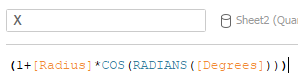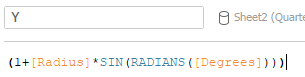Drag X to rows and Y to columns and change the aggregation to either MIN or MAX. Usually X would go to columns and Y would go to rows, but remember this had to be flipped in order to orient 0 and 360 degrees at the top of the pentagon. For this dashboard, I want to display a series of charts containing results for all categories. Therefore, I will create a separate chart for each category. To make life easier, I pick one category to start off with and filter on that category. Once this category’s chart is perfect, I can simply duplicate the sheet and change the filter to create charts for the other categories.

Change the Mark type to Line and bring ‘Player’ onto both the Label and Path cards. The line may look jumbled at first. The path must be sorted correctly to fix this and to eliminate the gap in the line. In large datasets it may help to add a rank column to your data to sort on.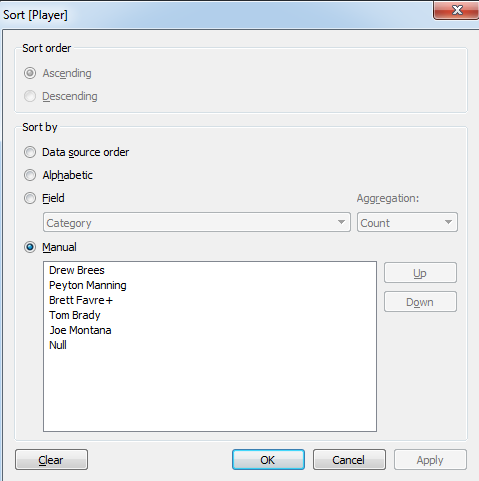Now for one very important part of the chart – the radar image! This can be created in Excel and brought into Tableau as a background image. Excel 2016 seems to have a slight bug where it will not create the spindles of the radar chart by default (the radial lines that connect the center to the edge of the shape). There is a workaround for this, though. Create a chart using the number of rows that matches how many dimensional values you are using. In this case we use 5 rows, since we are making a pentagon. You can pick Radar With Markers asthe chart type. In the chart formatting select Radar (Value) Axis. Then under Line, select Solid Line. Click Design on the ribbon and pick Change Chart Type, then select Radar. When you change this the radial lines should appear. Remove labels, change line fill to none, and remove the chart title. You want to be left with a blank chart. You can change the axis and background coloring to match your dashboard if desired. Screenshot the chart and bring it into PowerPoint to crop the image closely to the edge of the axis. Right click on the image to save as a picture. Now, we’re ready to import the image into Tableau.

Here is where we left off in Tableau:In the top menu bar of Tableau select the Map menu then Background Images. Pick the applicable data source. Click add image. You can then browse for the image that was just saved from PowerPoint. In drop downs on the left side of the window, select whatever is in Columns for X and whatever is in Rows for Y. Enter left/right and bottom/top bounds. For a circular shape, if you cropped the image closely the bounds can be 0, 2 for both X and Y. That is because the shape will extend to 2 on both axes. Remember, the center is at 1,1 and there is a radius of 1 to the edge. For the pentagon, 0/2 for bounds does not work because the bottom edge of this shape does not extend to where the edge of a circle would be. See from the below image that the bottom of the pentagon only extends down to 0.2 and not 0. In some cases, you will have to play around with the numbers to get the points to line up perfectly. Use the min/max values for reference. You know that the min value should be exactly on the center of the image and the max value should be on the outer edge.Once this is done, make final formatting changes such as removing halo from color, adding something to size/color on the marks card, removing zero lines, changing the sheet background color, and unchecking show header for the x and y axis. Get the plot area zoomed to your liking, then in Map Options uncheck ‘Allow Pan and Zoom’ and ‘Show View Toolbar’. If you plan to use tooltips, the duplicated coordinate we had to add to connect the line may interfere. To fix this, you can make a calculated field to use in the tooltip such as IF ISNULL([Player]) then 'Drew Brees' else [Player] END. Labels must be dragged into position manually. Duplicate the sheet and swap out the category filter to create the rest of the charts.

Here is the final product:## Bonus! Dynamic Spiral-Spider ChartThis spiral chart has all calculations done in Tableau with no extra data prep necessary (assuming your data follows standard best practices). The data I used here is the Digital Economy and Society Index (DESI) data from a recent Makeover Monday topic.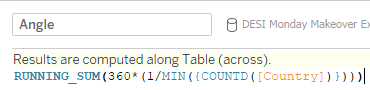In this chart, the first and last coordinate do not connect. Therefore, we can calculate the angle (degrees) in Tableau using a Table Calculation. Since there are 29 countries in the data, the end goal for the formula is to have (N1-N29 divided by 29)*360. Originally, it was my plan to use Index() to assign each country a number value of 1-29. However, I came across a blog post from The Data School in which they used Running Sum for a similarapplication. Running Sum is a bit easier to deal with for this. ‘Angle’ is what we will use to dynamically sort the spider. Radius, X, and Y calculated fields are the same as what I used earlier. To make this more dynamic, though, for Radius I am using a parameterized calculated field so that I can

toggle between metrics. I named this parameter ‘Select Indicator’. Since there are five unique ‘Indicators’ in this data, I created a unique measure for each of them using an IF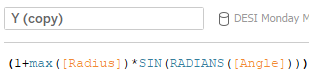statement as well as a measure for overall average. Then, I created a CASE calculation using a String List parameter.The Radius formula is the same, but with Select Indicator used to define it.Bring X to columns and Y to rows. In this dynamic example, Angle is placed on Path. For the chart to come together we need to set the ‘Compute Using’ option on the table calc for X, Y, and Angle to the same thing. I chose for these to be sorted on ‘Select Indicator’ so that when I toggle the parameter the chart will sort automatically. Note that you do not need a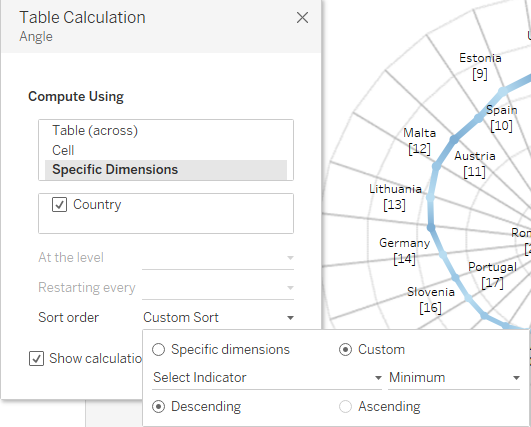parameter to make this dynamic. You can choose a measure to sort on, but whatever is chosen for the table calculation sort has to match what you used for Radius. Also, if you want, you could change to a clockwise spiral by sorting ascending instead.

This is the final look of the sheet after everything is set. With the Year filter added, any Year/Indicator combination can be selected and the chart will dynamically sort the countries.Author: Ben Steckler

bottom of page